Скачать презентацию CHAPTER 10 The Cost of Capital n n

d441bd6649cbe2f0cf6cca1d59e0cdb1.ppt

• Количество слайдов: 26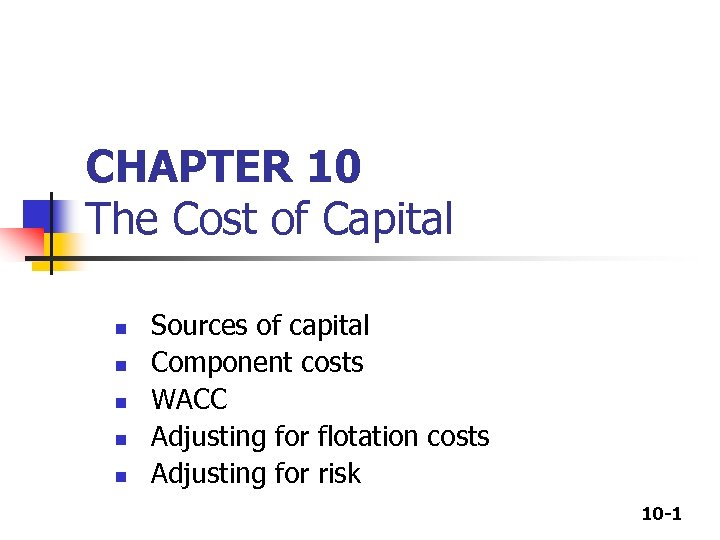CHAPTER 10 The Cost of Capital n n n Sources of capital Component costs WACC Adjusting for flotation costs Adjusting for risk 10 -1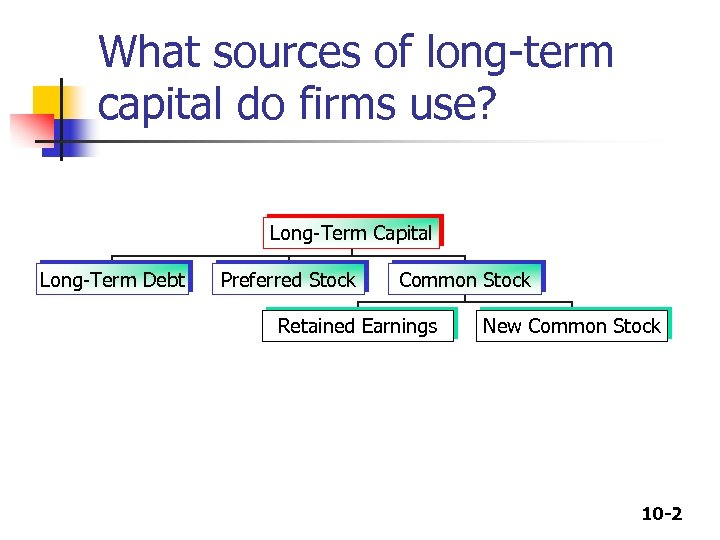What sources of long-term capital do firms use? Long-Term Capital Long-Term Debt Preferred Stock Common Stock Retained Earnings New Common Stock 10 -2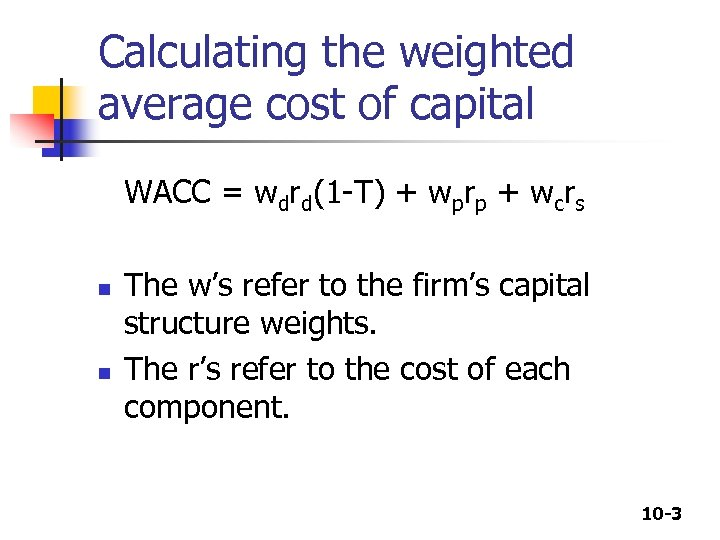Calculating the weighted average cost of capital WACC = wdrd(1 -T) + wprp + wcrs n n The w’s refer to the firm’s capital structure weights. The r’s refer to the cost of each component. 10 -3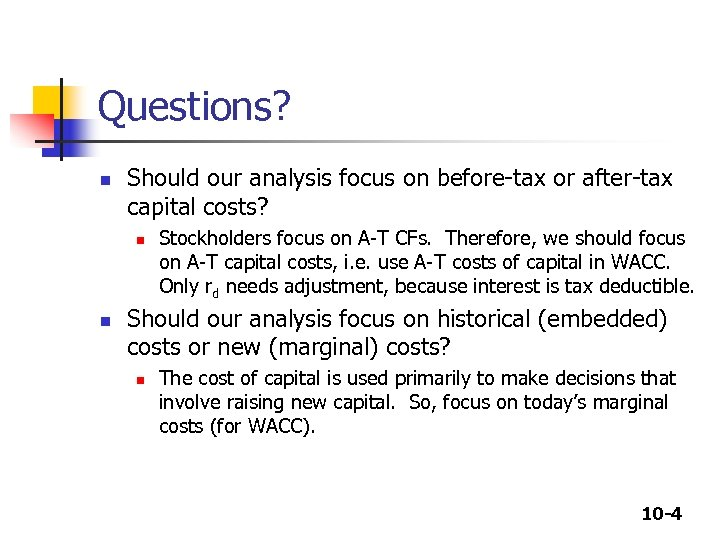Questions? n Should our analysis focus on before-tax or after-tax capital costs? n n Stockholders focus on A-T CFs. Therefore, we should focus on A-T capital costs, i. e. use A-T costs of capital in WACC. Only rd needs adjustment, because interest is tax deductible. Should our analysis focus on historical (embedded) costs or new (marginal) costs? n The cost of capital is used primarily to make decisions that involve raising new capital. So, focus on today’s marginal costs (for WACC). 10 -4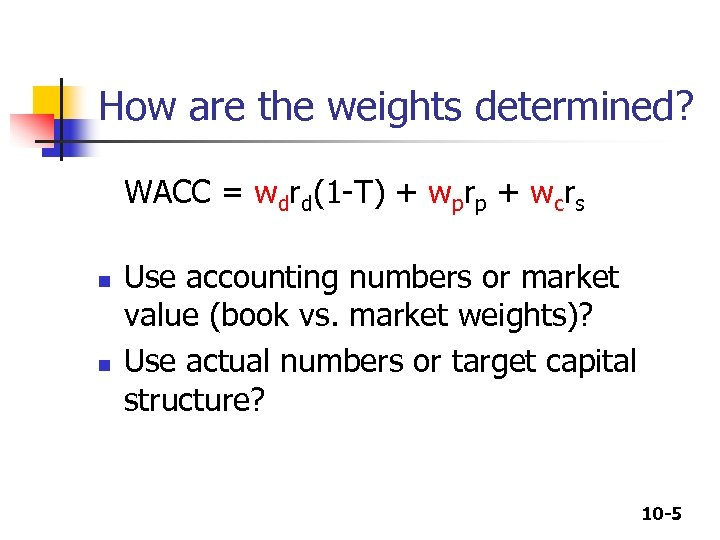How are the weights determined? WACC = wdrd(1 -T) + wprp + wcrs n n Use accounting numbers or market value (book vs. market weights)? Use actual numbers or target capital structure? 10 -5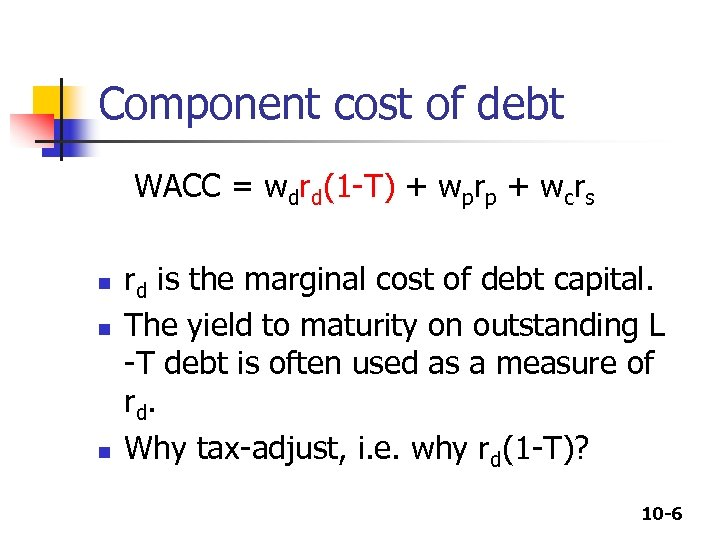Component cost of debt WACC = wdrd(1 -T) + wprp + wcrs n n n rd is the marginal cost of debt capital. The yield to maturity on outstanding L -T debt is often used as a measure of rd. Why tax-adjust, i. e. why rd(1 -T)? 10 -6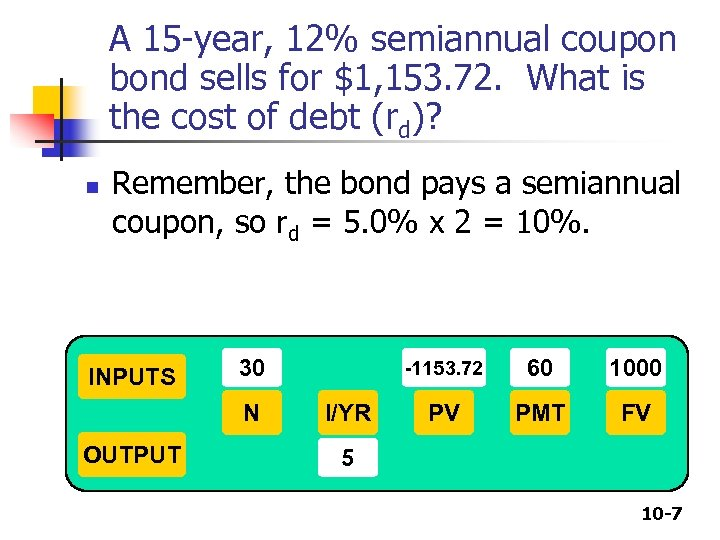A 15 -year, 12% semiannual coupon bond sells for \$1, 153. 72. What is the cost of debt (rd)? n Remember, the bond pays a semiannual coupon, so rd = 5. 0% x 2 = 10%. INPUTS 30 N OUTPUT -1153. 72 I/YR 60 1000 PV PMT FV 5 10 -7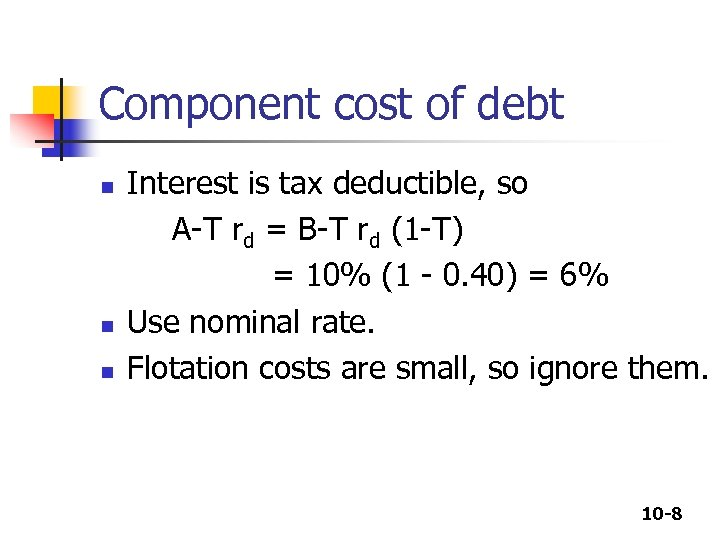Component cost of debt n n n Interest is tax deductible, so A-T rd = B-T rd (1 -T) = 10% (1 - 0. 40) = 6% Use nominal rate. Flotation costs are small, so ignore them. 10 -8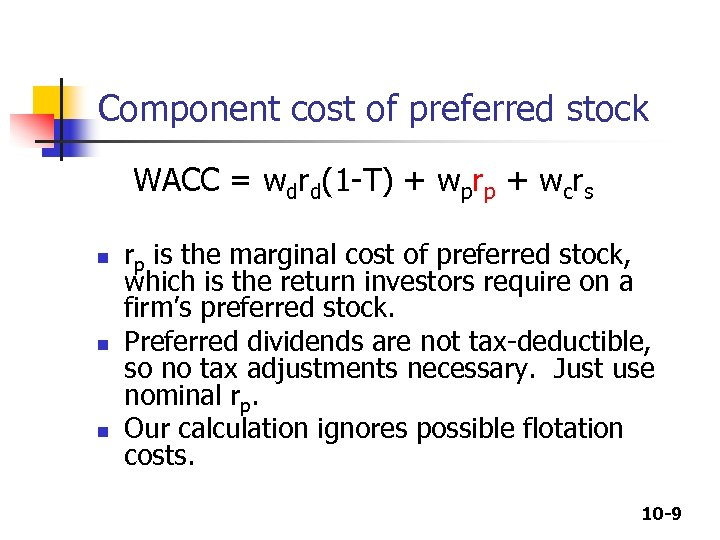Component cost of preferred stock WACC = wdrd(1 -T) + wprp + wcrs n n n rp is the marginal cost of preferred stock, which is the return investors require on a firm’s preferred stock. Preferred dividends are not tax-deductible, so no tax adjustments necessary. Just use nominal rp. Our calculation ignores possible flotation costs. 10 -9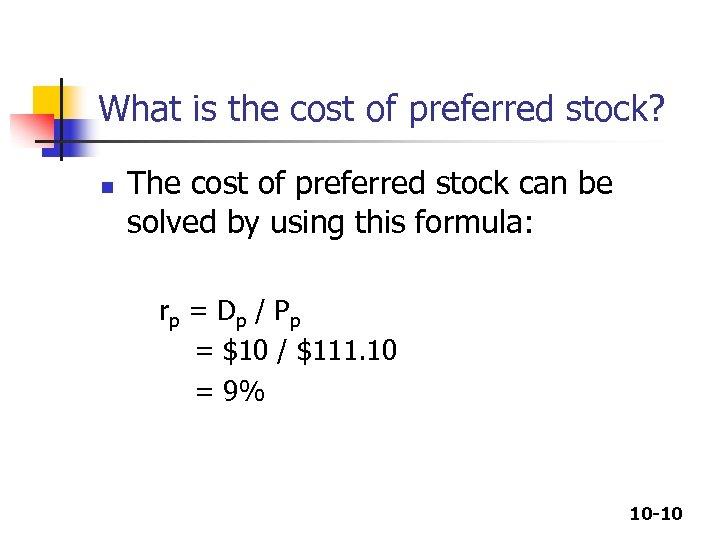What is the cost of preferred stock? n The cost of preferred stock can be solved by using this formula: rp = D p / P p = \$10 / \$111. 10 = 9% 10 -10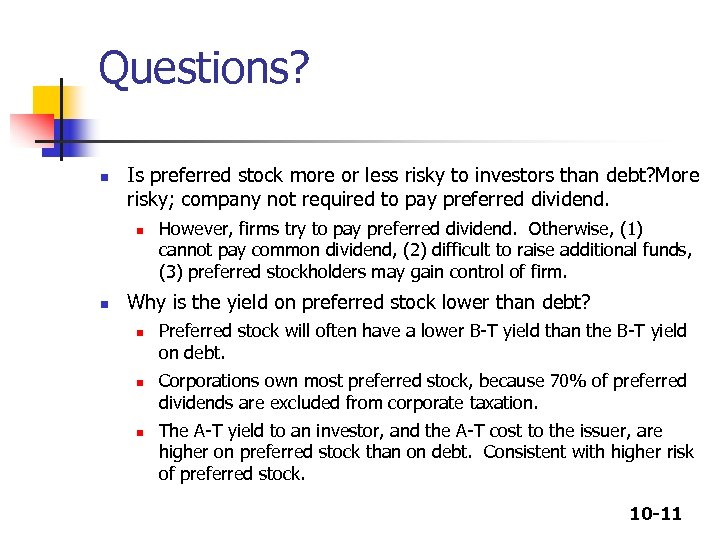Questions? n Is preferred stock more or less risky to investors than debt? More risky; company not required to pay preferred dividend. n n However, firms try to pay preferred dividend. Otherwise, (1) cannot pay common dividend, (2) difficult to raise additional funds, (3) preferred stockholders may gain control of firm. Why is the yield on preferred stock lower than debt? n n n Preferred stock will often have a lower B-T yield than the B-T yield on debt. Corporations own most preferred stock, because 70% of preferred dividends are excluded from corporate taxation. The A-T yield to an investor, and the A-T cost to the issuer, are higher on preferred stock than on debt. Consistent with higher risk of preferred stock. 10 -11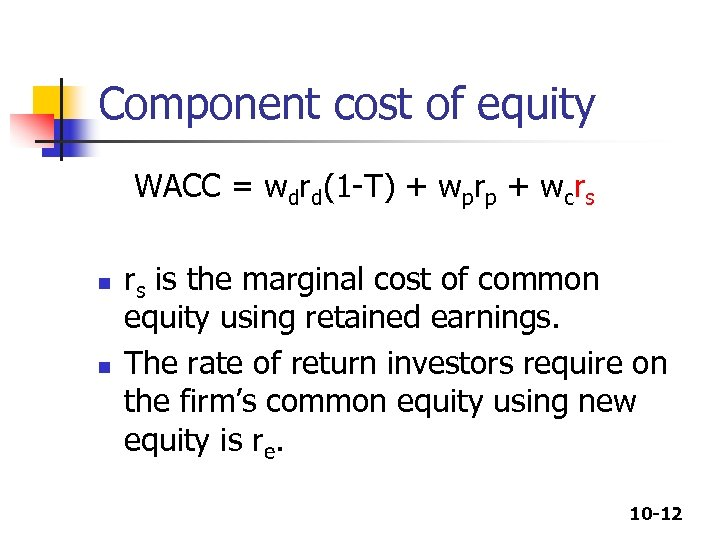Component cost of equity WACC = wdrd(1 -T) + wprp + wcrs n n rs is the marginal cost of common equity using retained earnings. The rate of return investors require on the firm’s common equity using new equity is re. 10 -12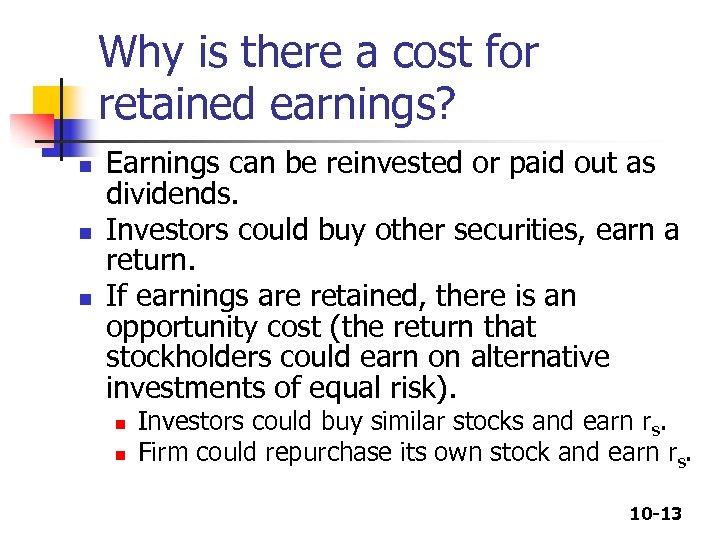Why is there a cost for retained earnings? n n n Earnings can be reinvested or paid out as dividends. Investors could buy other securities, earn a return. If earnings are retained, there is an opportunity cost (the return that stockholders could earn on alternative investments of equal risk). n n Investors could buy similar stocks and earn rs. Firm could repurchase its own stock and earn rs. 10 -13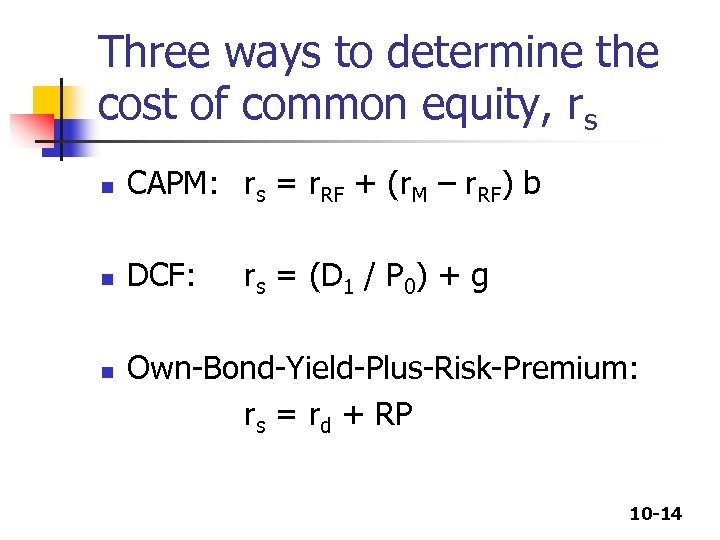Three ways to determine the cost of common equity, rs n CAPM: rs = r. RF + (r. M – r. RF) b n DCF: n rs = (D 1 / P 0) + g Own-Bond-Yield-Plus-Risk-Premium: rs = rd + RP 10 -14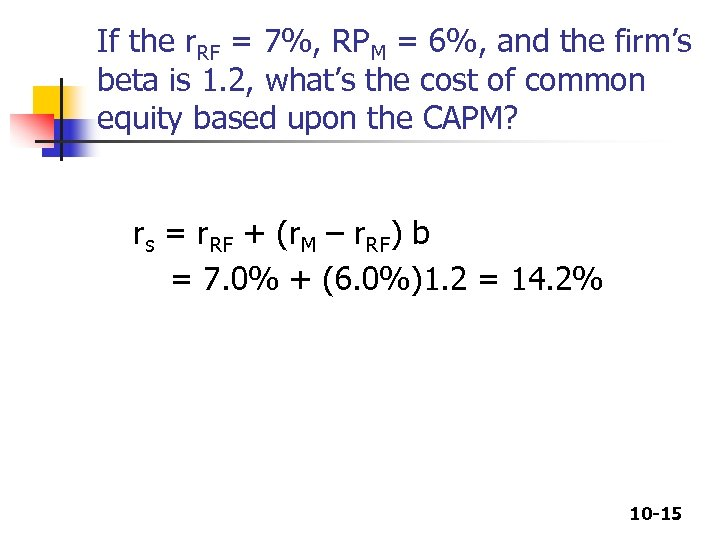If the r. RF = 7%, RPM = 6%, and the firm’s beta is 1. 2, what’s the cost of common equity based upon the CAPM? rs = r. RF + (r. M – r. RF) b = 7. 0% + (6. 0%)1. 2 = 14. 2% 10 -15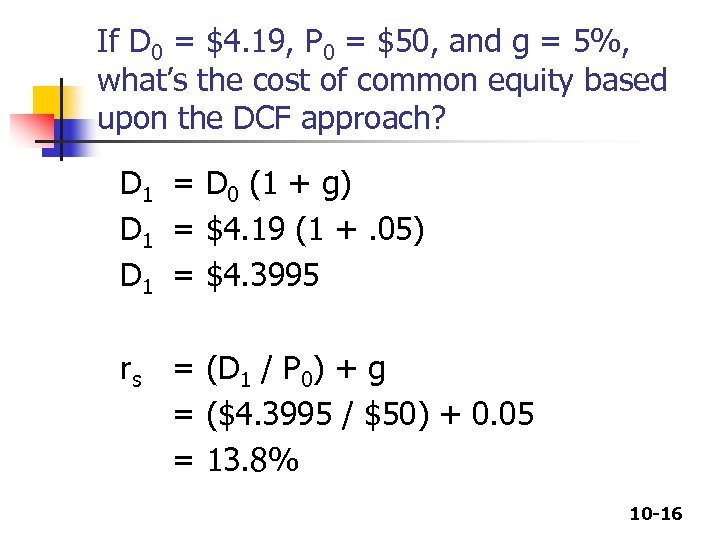If D 0 = \$4. 19, P 0 = \$50, and g = 5%, what’s the cost of common equity based upon the DCF approach? D 1 = D 0 (1 + g) D 1 = \$4. 19 (1 +. 05) D 1 = \$4. 3995 rs = (D 1 / P 0) + g = (\$4. 3995 / \$50) + 0. 05 = 13. 8% 10 -16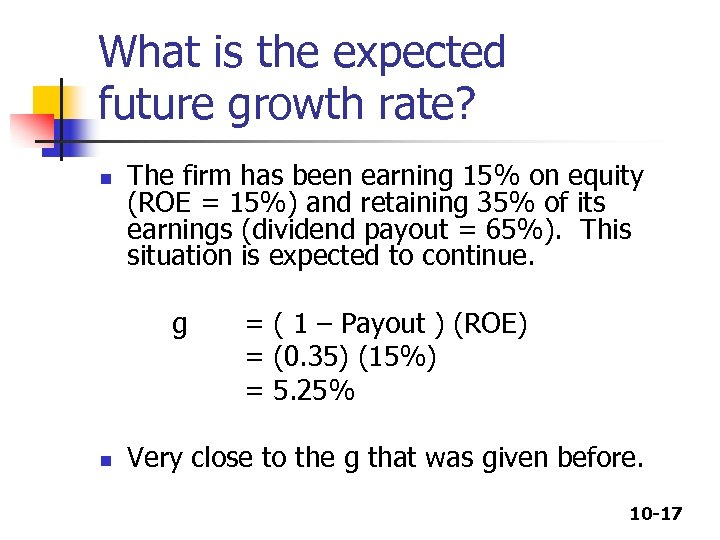What is the expected future growth rate? n The firm has been earning 15% on equity (ROE = 15%) and retaining 35% of its earnings (dividend payout = 65%). This situation is expected to continue. g n = ( 1 – Payout ) (ROE) = (0. 35) (15%) = 5. 25% Very close to the g that was given before. 10 -17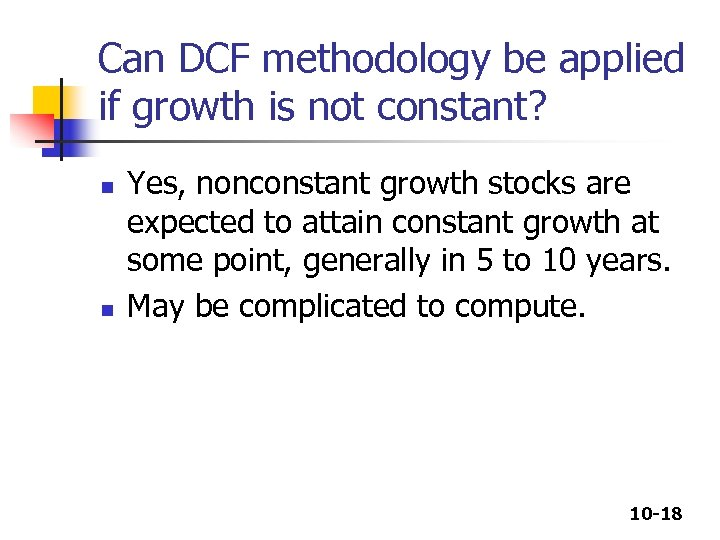Can DCF methodology be applied if growth is not constant? n n Yes, nonconstant growth stocks are expected to attain constant growth at some point, generally in 5 to 10 years. May be complicated to compute. 10 -18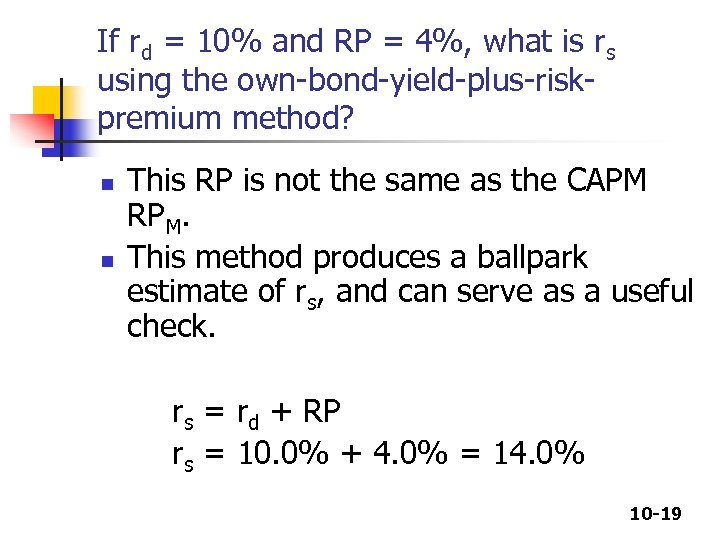If rd = 10% and RP = 4%, what is rs using the own-bond-yield-plus-riskpremium method? n n This RP is not the same as the CAPM RPM. This method produces a ballpark estimate of rs, and can serve as a useful check. rs = rd + RP rs = 10. 0% + 4. 0% = 14. 0% 10 -19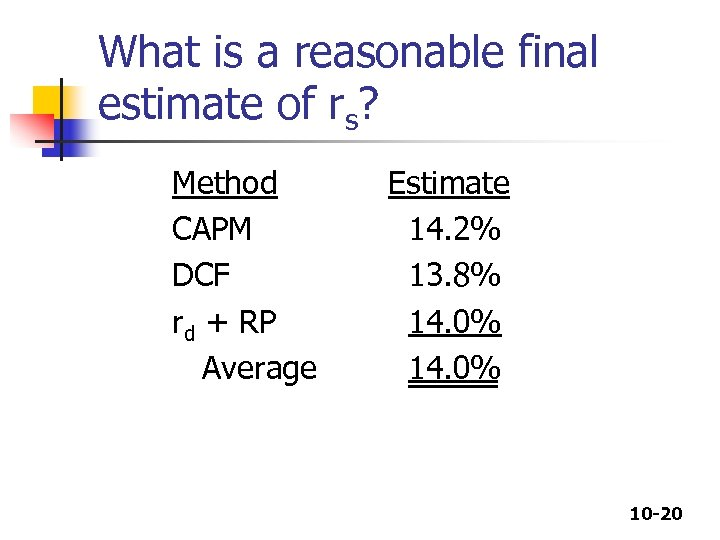What is a reasonable final estimate of rs? Method CAPM DCF rd + RP Average Estimate 14. 2% 13. 8% 14. 0% 10 -20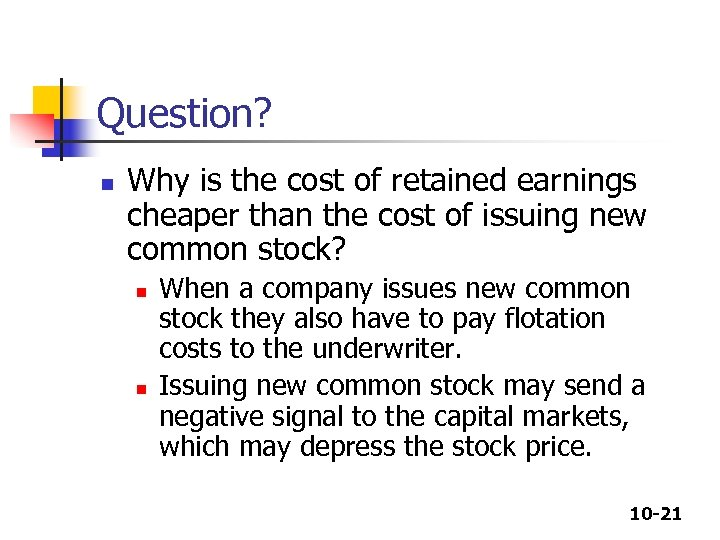Question? n Why is the cost of retained earnings cheaper than the cost of issuing new common stock? n n When a company issues new common stock they also have to pay flotation costs to the underwriter. Issuing new common stock may send a negative signal to the capital markets, which may depress the stock price. 10 -21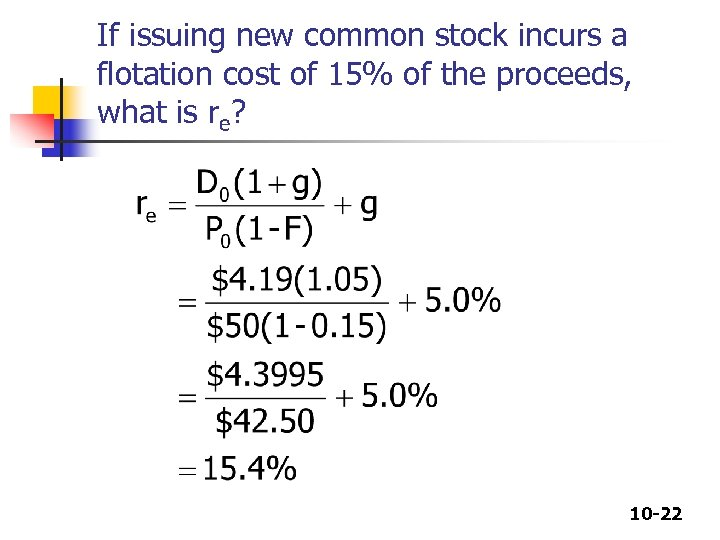If issuing new common stock incurs a flotation cost of 15% of the proceeds, what is re? 10 -22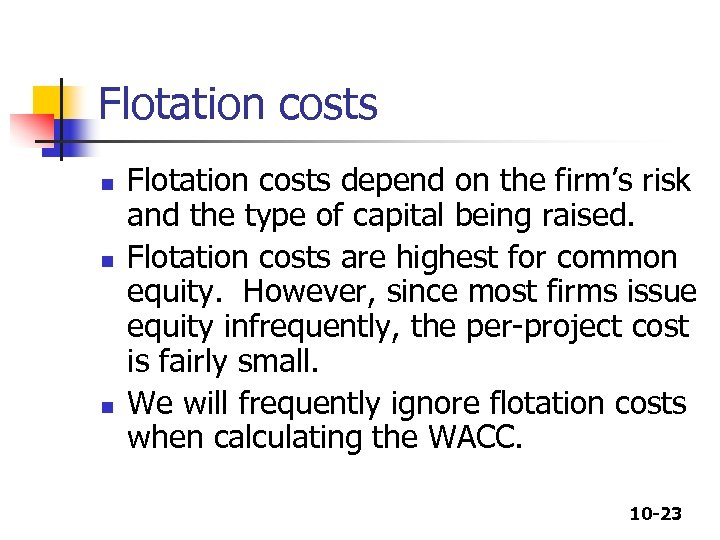Flotation costs n n n Flotation costs depend on the firm’s risk and the type of capital being raised. Flotation costs are highest for common equity. However, since most firms issue equity infrequently, the per-project cost is fairly small. We will frequently ignore flotation costs when calculating the WACC. 10 -23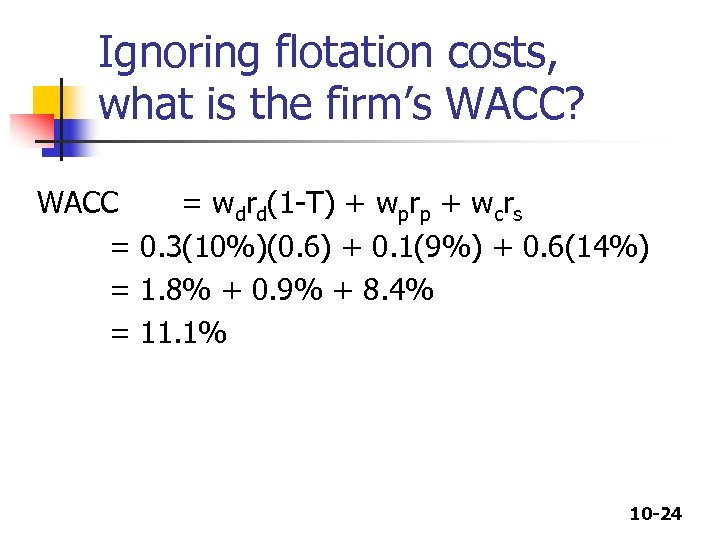Ignoring flotation costs, what is the firm’s WACC? WACC = wdrd(1 -T) + wprp + wcrs = 0. 3(10%)(0. 6) + 0. 1(9%) + 0. 6(14%) = 1. 8% + 0. 9% + 8. 4% = 11. 1% 10 -24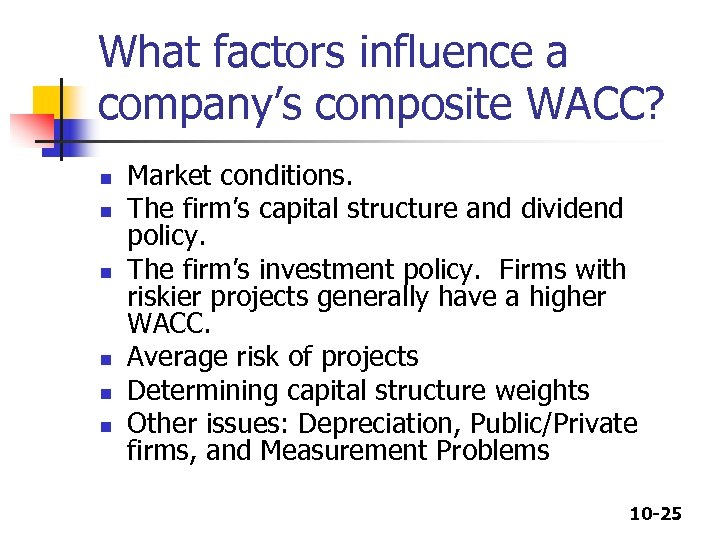What factors influence a company’s composite WACC? n n n Market conditions. The firm’s capital structure and dividend policy. The firm’s investment policy. Firms with riskier projects generally have a higher WACC. Average risk of projects Determining capital structure weights Other issues: Depreciation, Public/Private firms, and Measurement Problems 10 -25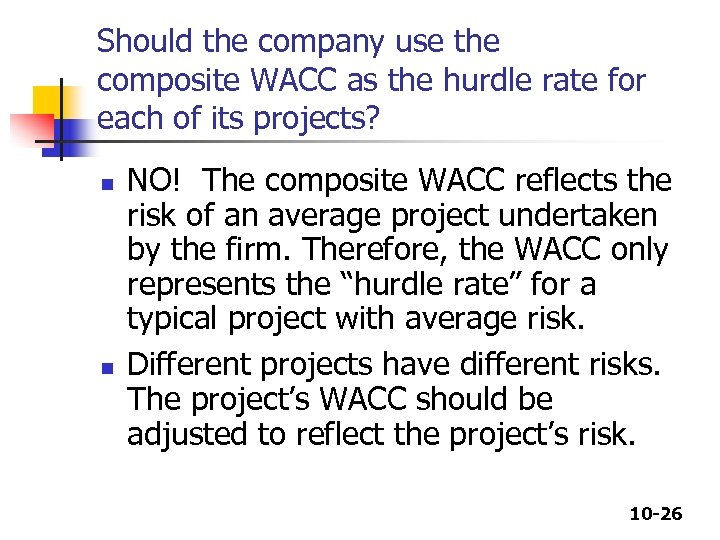Should the company use the composite WACC as the hurdle rate for each of its projects? n n NO! The composite WACC reflects the risk of an average project undertaken by the firm. Therefore, the WACC only represents the “hurdle rate” for a typical project with average risk. Different projects have different risks. The project’s WACC should be adjusted to reflect the project’s risk. 10 -26